Abstract: 本文继续上文的离散随机变量的观点，将部分试验模型转换到连续随机变量的观点上。
Keywords: Continuous Random Variable，Continuous Distributions，Probability Desity Function，Uniform Distributions on Intervals

# 连续分布

## 概率密度函数 The Probability Density Function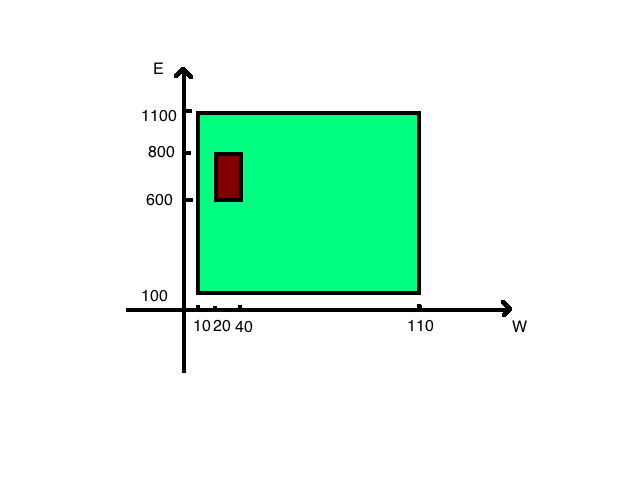### 连续分布，连续随机变量 Continuous Distribution & Continuous Random Variable

Definition Continuous Distribution/Random Variable: We say that a random variable $X$ has a continuous distribution variable if there exists a nonnegative function $f$ ,defined on the real line,such taht for every interval of real nombers (bounded or unbounded),the probability that $X$ takes a value in the interval is the integral of $f$ over the interval

$$Pr(a\leq X\leq b)=\int^b_af(x)dx$$

### 概率密度函数的定义 Definition Probability Density Function/p.d.f/Support

Definition Probability Density Function/p.d.f/Support:If $X$ has a continuous distribution ,the function $f$ described in definition Continuous Distribution/Random Variable is called the probability density function(abbreviated p.d.f)of $X$.The closure of the set ${x:f(x)>0}$ is called the support of (the distribution of) $X$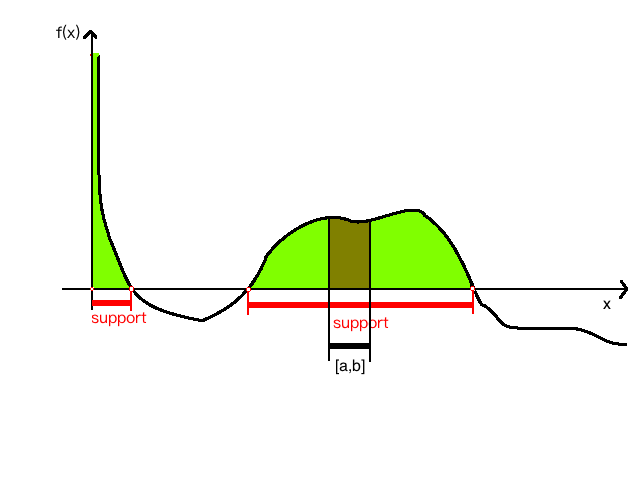pdf需要满足以下两点性质：

$$f(x)\geq 0 \quad \text{for all } x$$

$$\int^\infty_{-\infty}f(x)=1$$

## 概率（密度）函数的不唯一性 Nonuniqueness of the p.d.f.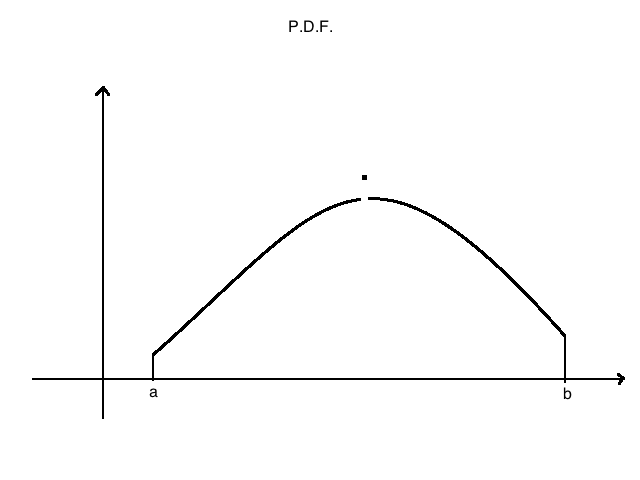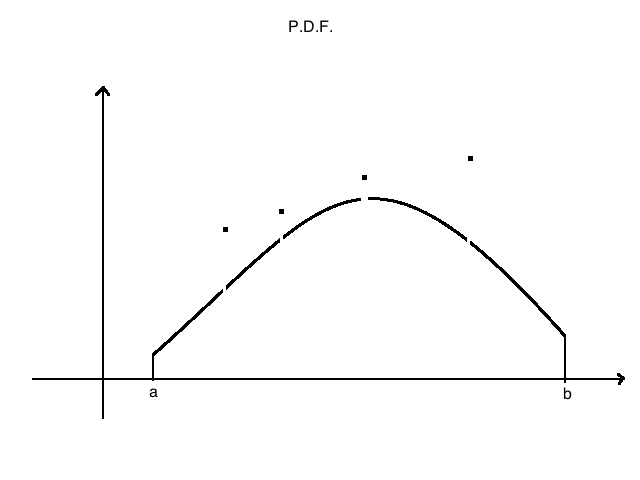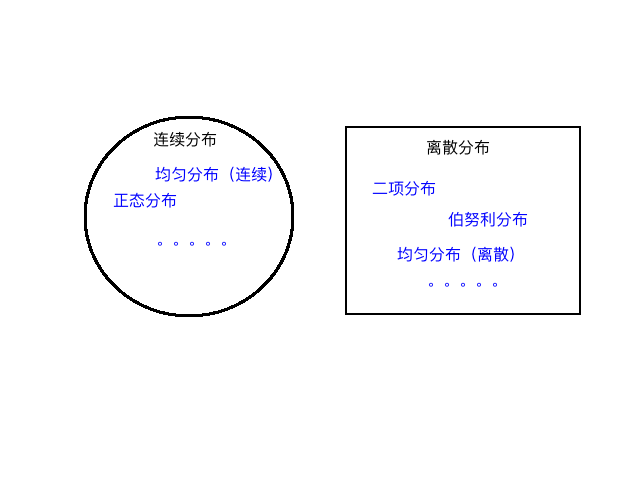## 区间上的均匀分布 Uniform Distributions on Intervals

Definition Uniform Distribution on an interval:Let $a$ and $b$ be two given real numbers such that $a<b$.Let $X$ be a random variable such that it is known that $a\leq X \leq b$ and ,for every subinterval of $[a,b]$ ,the probability that $X$ will belong to that subinterval is proportional to the length of that subinterval .We then say that the random variable X has the uniform distribution on the interval $[a,b]$

Theorem Uniform Distribution p.d.f. If X has the uniform distribution on an interval $[a,b]$,then the p.d.f. of X is:

$$f(x)= \begin{cases} \frac{1}{b-a} \quad \text{for} a\leq x\leq b\\ 0\quad\quad \text{otherwise} \end{cases}$$

$$\frac{\int^{x_2}_{x_1}f(x)dx}{\int^{b}_{a}f(x)dx}=\frac{|x_2-x_1|}{b-a}\quad \text{for:} x_2>x_1$$

QED

$$f(x)= \begin{cases} \frac{x}{8}\quad \text{for} 0<x<4\\ 0\quad \quad \text{otherwise} \end{cases}$$

$$Pr(1\leq X\leq 2)=\int^2_1\frac{1}{8}xdx=\frac{3}{16}$$

$$Pr(X>2)=\int^4_2\frac{1}{8}xdx=\frac{3}{4}$$

0%